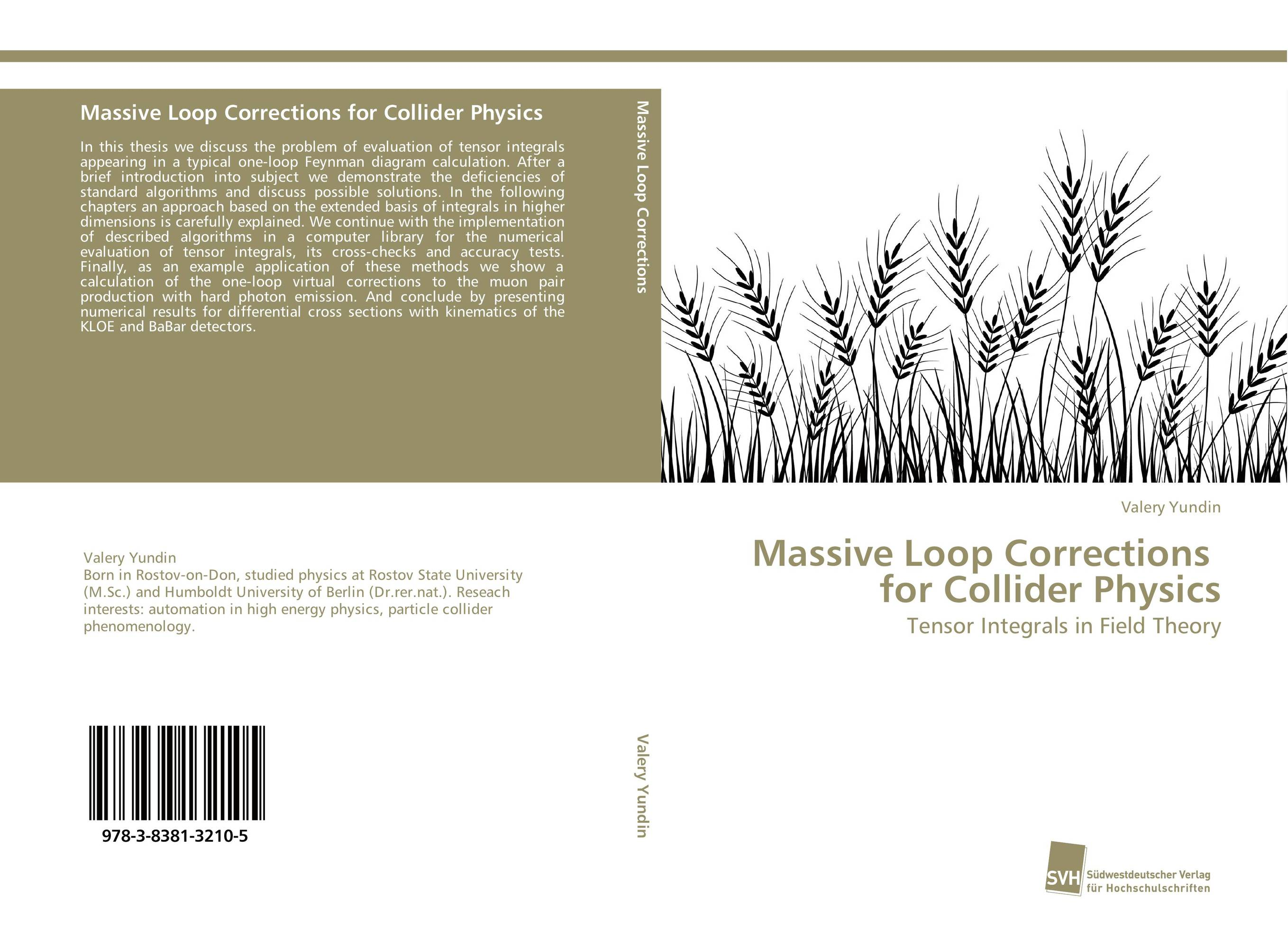# Massive Loop Corrections for Collider Physics

###### Аннотация:
In this thesis we discuss the problem of evaluation of tensor integrals appearing in a typical one-loop Feynman diagram calculation. After a brief introduction into subject we demonstrate the deficiencies of standard algorithms and discuss possible solutions. In the following chapters an approach based on the extended basis of integrals in higher dimensions is carefully explained. We continue with the implementation of described algorithms in a computer library for the numerical evaluation of tensor integrals, its cross-checks and accuracy tests. Finally, as an example application of these methods we show a calculation of the one-loop virtual corrections to the muon pair production with hard photon emission. And conclude by presenting numerical results for differential cross sections with kinematics of the KLOE and BaBar detectors.
###### Издания произведения:9783838132105

### Рекомендуем

#### Комментарии

Пока нет ни одного комментария
Вы должны войти для того что бы оставлять комментарии